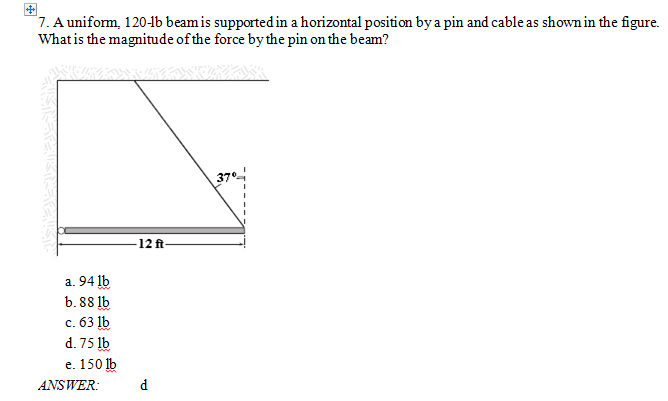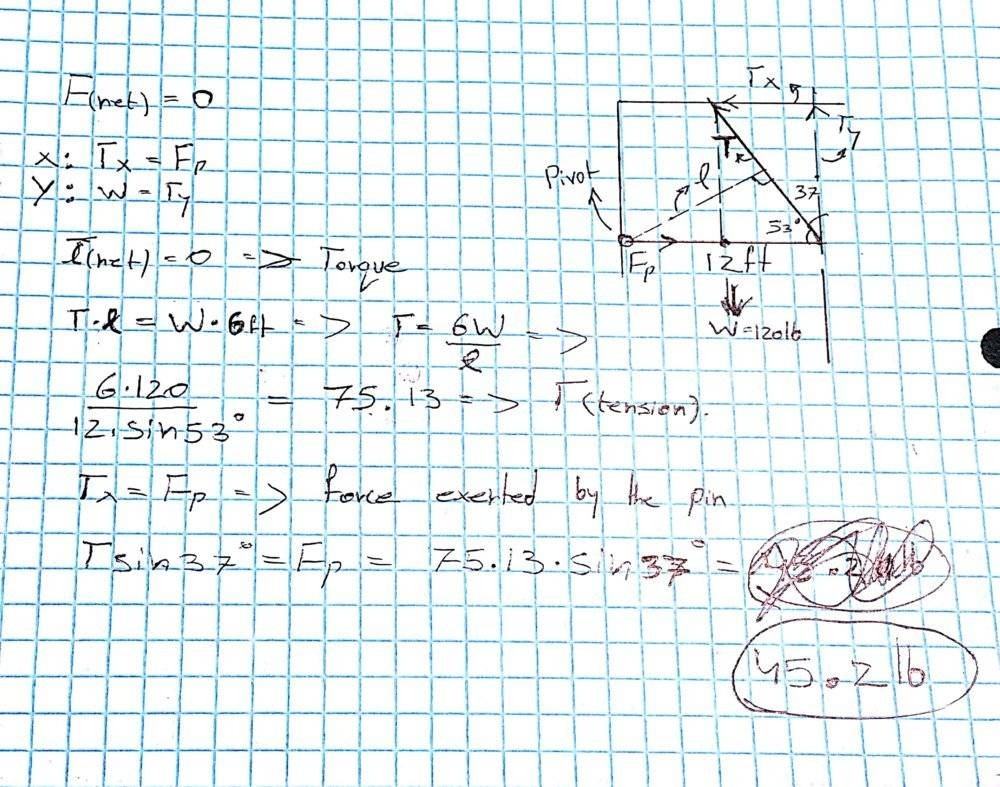# Static Equilibrium and a beam

I have been trying to work on this problem, but I keep getting the answer wrong. I would appreciate if someone could help me understand what I did wrong.Below is my answer and solution:#### Attachments

Last edited:

BvU
Homework Helper
How can you work with ft and lb and come up with a force in N ?

What is the direction of Fp ? You seem to think it's horizontal. Why ?

It's actually in lbs. I don't know why I wrote 45.2N. The answer is supposed to be in lbs.
And about the direction of F(p), I assumed it would be horizontal since the beam is in static equilibrium while remaining horizontally.

Chestermiller
Mentor
Why not just write the moment balance simply as $$(120)(6)=T_y(12)$$

Why not just write the moment balance simply as $$(120)(6)=T_y(12)$$
Wouldn't the answer still be the same?

BvU
Homework Helper
I assumed it would be horizontal since the beam is in static equilibrium
Assumption is unfounded.

Assumption is unfounded.
Then what would be the correct answer?

Chestermiller
Mentor
Wouldn't the answer still be the same?
Yes, but wouldn’t be simpler? This would also allow you to immediately determine the vertical reaction force component at the pin.

jack action
Gold Member
But you have the 75 lb right there in your calculations.

The question is what is the magnitude of the force, not what is the horizontal component of the force.

Last edited:
haruspex
Homework Helper
Gold Member
2020 Award
But you have the 75 lb right there in your calculations.

The question is what is the magnitude of the force, not what is the horizontal component of the force.
Not exactly. The 75lb found in post #1 is for the tension in the cable. However, there is a neat way of seeing that the reaction at the hinge must have the same magnitude.
And about the direction of F(p), I assumed it would be horizontal
Easily falsified. Consider moments about the tip of the beam.

•Chestermiller